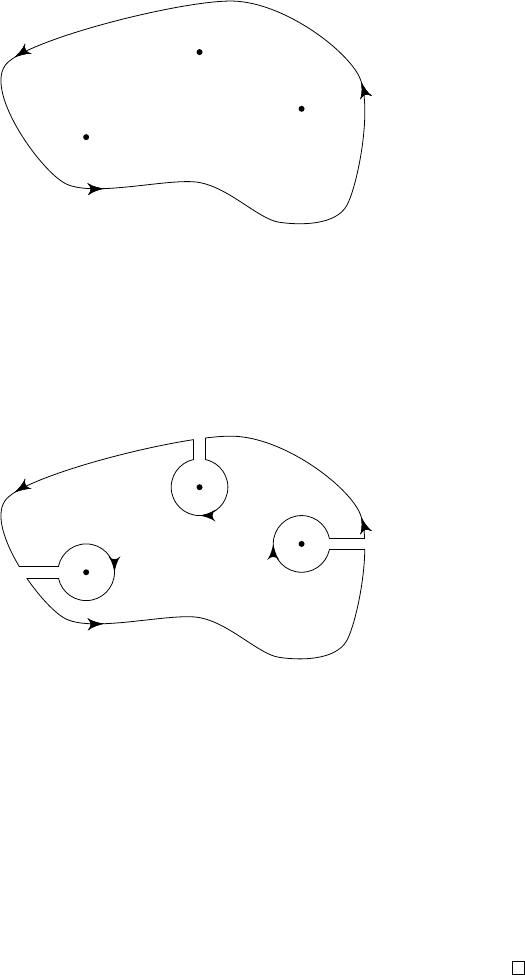4The calculus of residues

IB Complex Methods4.1 The residue theorem
We are now going to massively generalize the last result we had in the previous
chapter. We are going consider a function
f
with many singularities, and obtain
an analogous formula.
Theorem
(Residue theorem)
.
Suppose
f
is analytic in a simply-connected
region except at a finite number of isolated singularities
z
1
, ··· , z
n
, and that a
simple closed contour γ encircles the singularities anticlockwise. Then
I
γ
f(z) dz = 2πi
n
X
k=1
res
z=z
k
f(z).
z
1
z
2
z
3
γ
Note that we have already proved the case
n
= 1 in the previous section. To
prove this, we just need a difficult drawing.
Proof.
Consider the following curve
ˆγ
, consisting of small clockwise circles
γ
1
, ··· , γ
n
around each singularity; cross cuts, which cancel in the limit as they
approach each other, in pairs; and the large outer curve (which is the same as
γ
in the limit).
ˆγ
z
1
z
2
z
3
Note that
ˆγ
encircles no singularities. So
H
ˆγ
f
(
z
) d
z
= 0 by Cauchy’s theorem.
So in the limit when the cross cuts cancel, we have
I
γ
f(z) dz +
n
X
k=1
I
γ
k
f(z) dz =
I
ˆγ
f(z) dz = 0.
But from what we did in the previous section, we know
I
γ
k
f(z) dz = 2πi res
z=z
k
f(z),
since
γ
k
encircles only one singularity, and we get a negative sign since
γ
k
is a
clockwise contour. Then the result follows.
This is the key practical result of this course. We are going to be using this
very extensively to compute integrals.